Math resources Statistics and probability

Averages and range

Mean median mode

# Mean, Median, Mode

Here you will learn about the measures of central tendency, which is the mean, median and mode of data sets, including what they are and how to find them.

Students first learn about measures of central tendency in the 6 th grade and expand this knowledge as they move through middle school and high school statistics.

## What is mean median mode?

The mean, median and mode are different measures of center of a numerical data set. They are a way of summarizing a data set with a single number.

### Mean

The mean is the average of a numerical data set.

To calculate the mean, find the total of the values and divide the total by the number of values.

The “number of values” is sometimes referred to as the “number of numbers”.

Let’s find the mean of this data set.

The mean is 4.57 (rounded to 2 decimal places)

Step-by-step guide: Mean in math

### Median

The median is the middle number of a numerical data set.

To find the median, we need to arrange the values in numerical order, from the smallest value to the highest value, and find the middle value.

The middle value is the median value.

Let’s find the median of the same data set.

The median is 5 because it is the middle number of data points.

Step-by-step guide: Median

### Mode

The mode is the most common number. To find the mode, we need to find the value in the data set that occurs the most number of times.

Let’s find the mode of the data set.

The number that occurs the most or is most frequent is 6.

The mode is 6.

Step-by-step guide: Mode in math

### Range

The range is the difference between the greatest value and the least value of a data set. It is a measure of variability not a measure of center.

Let’s find the range of the data set.

7 - 1 = 6

The range is 6.

Step-by-step guide: Range in math

### What is mean median mode?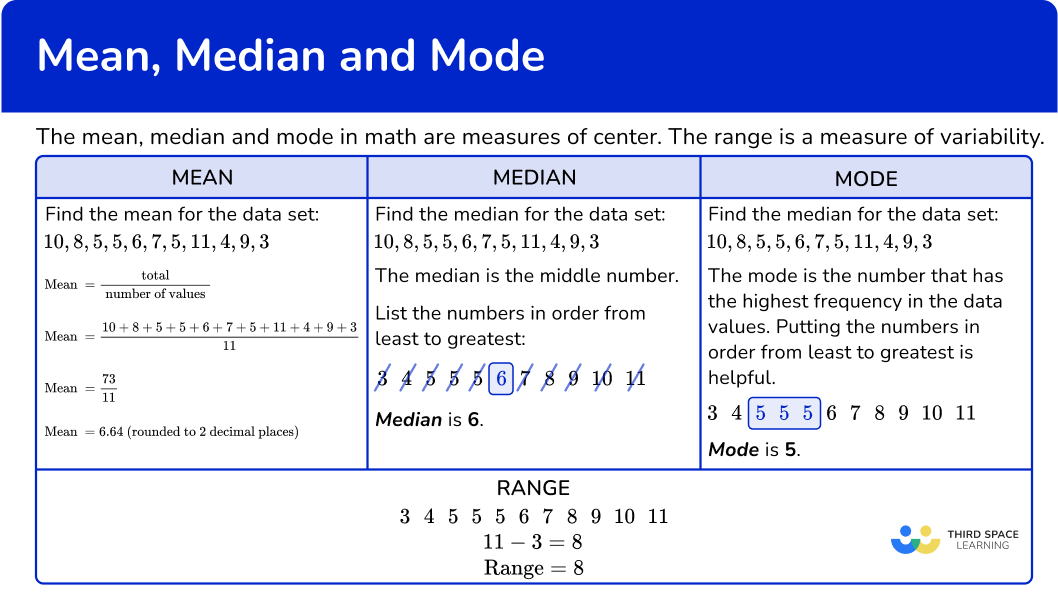## Common Core State Standards

How does this apply to 6 th grade math?

• Grade 6 – Statistics and Probability 6.SP.A.3
Recognize that a measure of center for a numerical data set summarizes all of its values with a single number, while a measure of variation describes how its values vary with a single number.

• Grade 6 – Statistics and Probability 6.SP.A2
Understand that a set of data collected to answer a statistical question has a distribution which can be described by its center, spread, and overall shape.

• Grade 6 – Statistics and Probability 6.SP.B.5c
Giving quantitative measures of center (median and/or mean) and variability (interquartile range and/or mean absolute deviation), as well as describing any overall pattern and any striking deviations from the overall pattern with reference to the context in which the data were gathered.

## How to find the mean median mode

In order to find the mean, median or mode:

1. List the numbers in order from least to greatest.
2. To find the mean, find the total of the numbers in the data set and divide by the number of values in the data set.
3. To find the median, find value(s) in the middle of the data set.
4. To find the mode, look for the value(s) that occur the most; there may be more than one mode.
5. To find the range, find the difference between the largest value and the smallest value.

## Mean median mode examples

### Example 1: finding the mode

Find the mode for the given data set.

1. List the numbers in order from least to greatest.

4To find the mode, look for the value(s) that occur the most; there may be more than one mode.

7 occurs the most, so the mode is 7.

### Example 2: finding the median

Find the median for the given data set.

List the numbers in order from least to greatest

To find the median, find values in the middle of the data set.

### Example 3: finding the median

Find the median of the given data set.

List the numbers in order from least to greatest.

### Example 4: finding the mean

Find the mean of the given data set.

List the numbers in order from least to greatest.

To find mean, find the total of the numbers in the data set and divide by the number of values in the data set.

### Example 5: finding the mean, median, and mode

Find the mean, median, and mode of the data set.

List the numbers in order from least to greatest.

To find the mean, find the total of the numbers in the data set and divide by the number of values in the data set.

To find the median, find values in the middle of the data set.

To find the mode, look for the value(s) that occur the most; there may be more than one mode.

### Example 6: finding the mean, median, mode, and range

Find the mean, median, and mode of the data set.

List the numbers in order from least to greatest.

To find the mean, find the total of the numbers in the data set and divide by the number of values in the data set.

To find the median, find values in the middle of the data set.

To find the mode, look for the value(s) that occur the most; there may be more than one mode.

To find the range, find the difference between the largest value and the smallest value.

### Teaching tips for mean, median, mode

• Incorporate project based data science learning activities where students have opportunity to collect their own data, as well as do data analysis/statistical analysis on the collected data.

• Although worksheets and paper-based quizzes have their place, consider alternate forms of formative assessments that engage students to gain a global perspective by having them summarize data in real time with real world data points.

### Easy mistakes to make

• Mixing up the measures of center
For example, if a student is asked to find the median and they find the mean.

• Listing the numbers in descending order instead of ascending order
When summarizing data, the best way to list the data is in ascending order (from least to greatest) not descending order (greatest to least).

• Thinking that the mean and median have to be a whole number
When summarizing data, the mean and median do not necessarily have to be whole numbers. For example, for this data set, the mean is not a whole number.

10, \, 8, \, 5, \, 5, \, 6, \, 7, \, 5, \, 11, \, 4, \, 9, \, 3

In this data set, the median is not a whole number.

12 and 13 are the middle numbers so to find the median, find the average between the two numbers.

Median = 12.5

### Practice mean median mode questions

1. Find the mode of the given data set.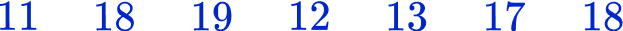12171816The value that occurs the most in the data set is 18. The other numbers only occur once.

2. Find the mode of the given data set.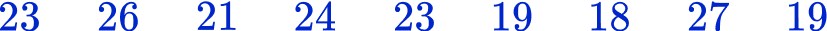19212319 and 23The most common values are 19 and 23. These two values occur twice within the data set. The other values only occur once.

3. Find the median of the given data set.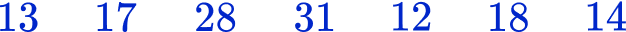18191731Put the numbers in order from the smallest number to largest number. Then find the middle value.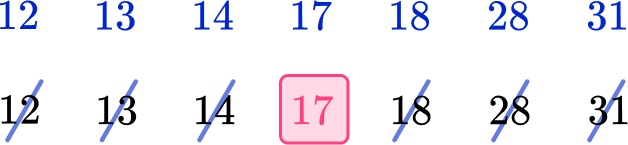The middle value is 17, so this is the median.

4. Find the median of the given data set.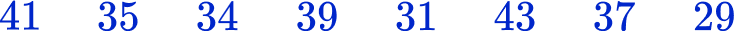35363738Put the numbers in order from the smallest to largest. Then find the middle value.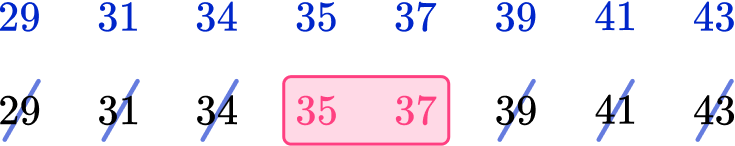The middle pairs of values are 35 and 37. The average of these is 36. The median is 36.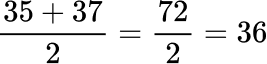5. Find the mean of the given data set.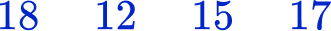1712.516.415.5To find the mean, add up all the values in the data set and divide by the number of values in the data set.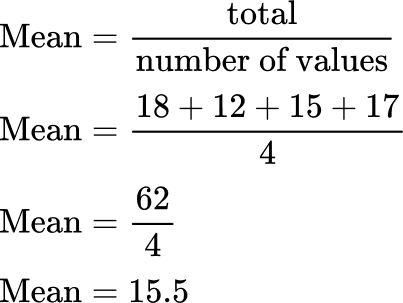6. Find the mean, median, mode, and range for the given data set (round to two decimal places when necessary).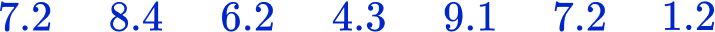Mean = 6.23, Median = 7.2, Mode = 7.2, Range = 7.9Mean = 6, Median = 6.2, Mode = 7.2, Range = 8Mean = 7, Median = 7.2, Mode = 7.2, Range = 7Mean = 6.2, Median = 7, Mode = 7, Range = 7.2List the numbers in order from least to greatest.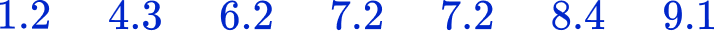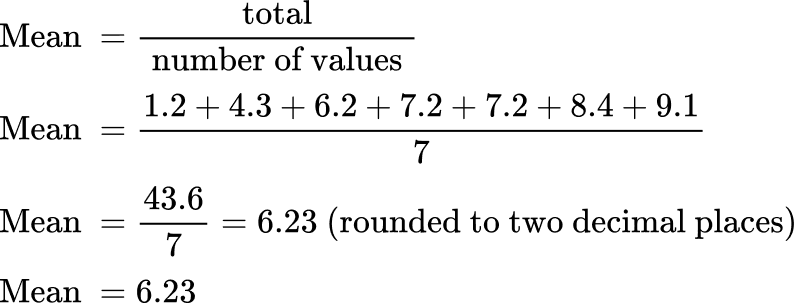The value in the middle is 7.2.

The median is 7.2.The value that occurs the most is 7.2. The mode is 7.2.

Range = 9.1-1.2 = 7.9

Range = 7.9

## Mean, median, mode FAQs

Can decimals and fractions be part of data sets?

Yes, data sets can be made up of whole numbers (odd numbers and even numbers), integers, decimals, and fractions.

What is the arithmetic mean?

The arithmetic mean is the mean (average value) where you add all the values and divide by the number of values in the set of data.

What is an outlier?

An outlier is an extremely high or low data point in relation to the rest of the set of numbers in the data set.

What is the geometric mean?

The geometric mean is the square root of a product of two numbers.

What is the standard deviation?

The standard deviation is a statistical measure that measures the dispersion of data points relative to the mean.

What is descriptive statistics?

Descriptive statistics is a method of summarizing data. Measures of central tendency are descriptive statistics.

## Still stuck?

At Third Space Learning, we specialize in helping teachers and school leaders to provide personalized math support for more of their students through high-quality, online one-on-one math tutoring delivered by subject experts.

Each week, our tutors support thousands of students who are at risk of not meeting their grade-level expectations, and help accelerate their progress and boost their confidence.